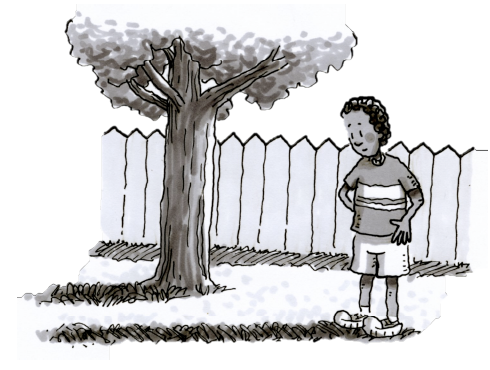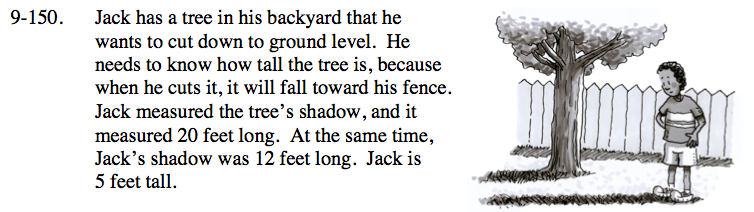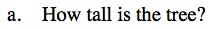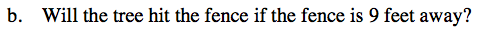Home > CC3 > Chapter 9 > Lesson 9.2.7 > Problem9-150

9-150.
1.Jack has a tree in his backyard that he wants to cut down to ground level. He needs to know how tall the tree is, because when he cuts it, it will fall toward his fence. Jack measured the tree’s shadow, and it measured 20 feet long. At the same time, Jack’s shadow was 12 feet long. Jack is 5 feet tall. Homework Help ✎

1. How tall is the tree?

2. Will the tree hit the fence if the fence is 9 feet away?Since the shadow lengths are proportional to their heights, we can create an equation using the given ratios.

Relate the shadow length to height ratios for the tree and for Jack and then solve for the unknown variable of the tree's height.

$\frac{20}{\it x} = \frac{12}{5}$

Tree's height = 8.33 ft.Based on the answer from part (a), was the tree's height taller than nine feet?